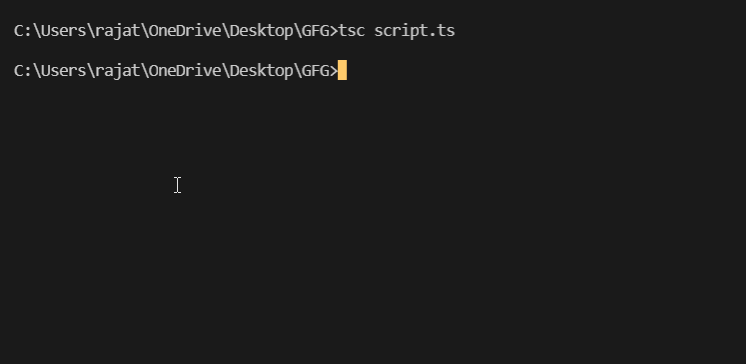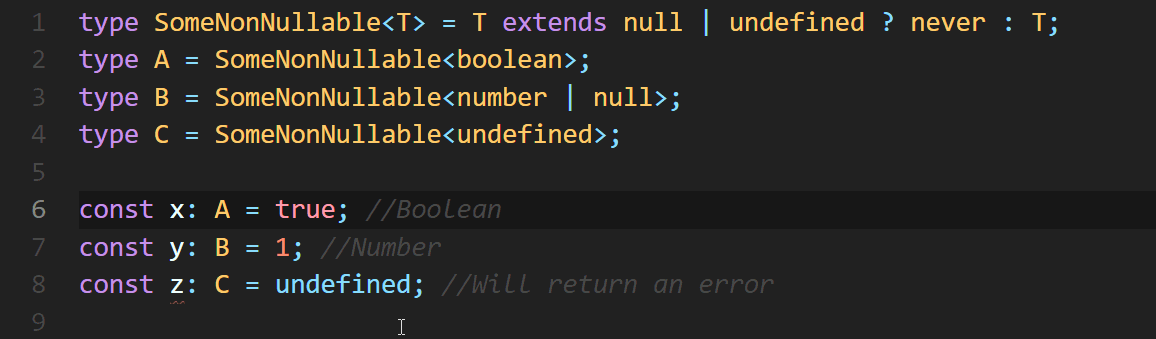Open in App
Not now

# Explain the purpose of never type in TypeScript

• Last Updated : 01 Feb, 2022

The never type represents a wide range of type of values that never occur. This means that it could be the return type of a function that never returns a particular value. In this article, we will learn about the never type in TypeScript and why it is used.

Syntax:

```// For variable
const GFG : never;

// For function
function geeks(): never {
const geeksForGeeks = 1;
}```

The following examples will illustrate the purpose or use of never type well:

Example 1: This example represents a return type of a function that never occurs. Due to the infinite loop, it is impossible to determine the return type.

## Javascript

 `// Function which executes an infinite  ` `// amount of times and never ends. ` ` `  `function` `infiniteLoop(): never{ ` `    ``while``(``true``){ ` `        ``console.log(``'GeekforGeeks'``); ` `    ``} ` `}`

Output:Since there is an infinite loop present in the program, it can crash the program or freeze your computer. Execution is automatically halted and no output is generated.

Example 2: This Example also represents a return type of a function that never occurs. But the difference is that the function is always throwing an error or an exception.

## Javascript

 `// Function always throwing an error/exception ` ` `  `function` `err(message: string): never{ ` `    ``throw` `Error(message); ` `} ` ` `  `err(``"My error!"``);`

Output:Example 3: Here, if the variable temp is neither a string nor a number, it has the never type assigned to it because TypeScript has determined there’s nothing left.

## Javascript

 `// Function demonstrating exhaustive checks ` `function` `f(temp: string | number): boolean { ` `    ``if` `(``typeof` `temp === ``"string"``) { ` `        ``return` `true``; ` `    ``} ``else` `if` `(``typeof` `temp === ``"number"``) { ` `        ``return` `false``; ` `    ``} ` ` `  `    ``// Not a string or a number. Value can't ` `    ``// occur here hence type is "never" ` `    ``return` `fail(``"Unexhaustive"``); ` `} ` ` `  `function` `fail(msg: string): never { ` `    ``throw` `new` `Error(msg); ` `} ` ` `  `console.log(f(23)); ``// Number ` `console.log(f(``"String"``)); ``// String`

Output:In every instance, since there are no defined return type annotations nor any reachable endpoints, TypeScript automatically infers the never type.

Apart from better type safety, as clearly demonstrated in the examples above, the never type has another common use case in the form of conditional types. Undesired types can be excluded from programs.

Example 4: The code snippet below gives an idea of this:

## Javascript

 `type SomeNonNullable = T extends ``null` `| undefined ? never : T; ` `type A = SomeNonNullable; ` `type B = SomeNonNullable; ` `type C = SomeNonNullable; ` `  `  `const x: A = ``true``; ``// Boolean ` `const y: B = 1; ``// Number ` `const z: C = undefined; ``// Will return an error `

Output:Here we are checking if T is null or undefined. If it is then we are pointing out that it should not be reachable (unreachable code).

My Personal Notes arrow_drop_up
Related Articles